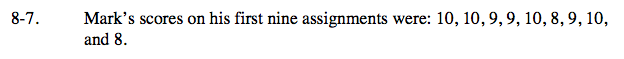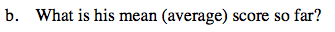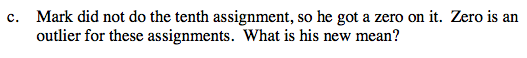### Home > CC1 > Chapter 8 > Lesson 8.1.1 > Problem8-7

8-7.
1. Mark’s scores on his first nine assignments were: 10, 10, 9, 9, 10, 8, 9, 10, and 8. Homework Help ✎

1. What are the median and range of his scores?

2. What is his mean (average) score so far?

3. Mark did not do the tenth assignment, so he got a zero on it. Zero is an outlier for these assignments. What is his new mean?To find the median arrange the scores in numerical order. Which one is in the middle of your list? The range is the difference between the highest and lowest values.Remember that the mean is the number which represents what every part would be if the total were divided evenly among the parts.

Add each part together to find the total.

10 + 10 + 9 + 9 + 10 + 8 + 9 + 10 + 8 = 83

Divide the total by the number of parts.

$\frac{83}{9}\approx9.2$This problem is very similar to part (b). However, you will need to add another test score (0).
Remember that this also means there is an additional part by which to divide.